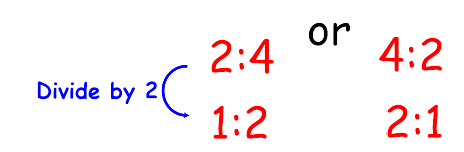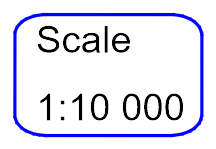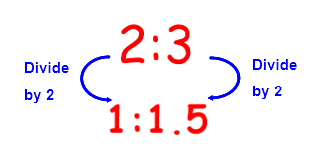# Express Ratio in the Form 1:n

In this worksheet, students will express a ratio in the form 1:nKey stage:  KS 3

Curriculum topic:   Ratio, Proportion and Rates of Change

Curriculum subtopic:   Use Ratio Notation

Difficulty level:#### Worksheet Overview

In this activity, we will simplify ratio where one of the parts of the ratio will have a value of 1.

For example,We call this type of simplifying 1:n (where n can be any number)

The reason we do this is because maps and scale drawings are usually given as a ratio of 1: another value.

It is a great way to compare data too!You may have seen the scale on a map written as:A typical question might look like this:

Example

Express 2:3 in the form 1:n

In this case, the left-hand side needs to be 1

As it is currently 2, we need to divide by 2 to make that 1

We must then do the same to the right-hand side.Therefore, we write the answer as 1:1.5

Don't worry that you have a decimal number in the ratio - this often happens!

Let's have a go at some questions now.You will need a calculator!

### What is EdPlace?

We're your National Curriculum aligned online education content provider helping each child succeed in English, maths and science from year 1 to GCSE. With an EdPlace account you’ll be able to track and measure progress, helping each child achieve their best. We build confidence and attainment by personalising each child’s learning at a level that suits them.

Get started